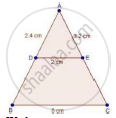Share

Books Shortlist
Your shortlist is empty

# Solution for In a δAbc, D and E Are Points on Ab and Ac Respectively Such that De || Bc. If Ad = 2.4cm, Ae = 3.2 Cm, De = 2cm and Bc = 5 Cm, Find Bd and Ce. - CBSE Class 10 - Mathematics

ConceptBasic Proportionality Theorem Or Thales Theorem

#### Question

In a ΔABC, D and E are points on AB and AC respectively such that DE || BC. If AD = 2.4cm, AE = 3.2 cm, DE = 2cm and BC = 5 cm, find BD and CE.

#### SolutionWe have,

DE || BC

Now, In ΔADE and ΔABC

∠A = ∠A [common]

∠ADE = ∠ABC [∵ DE || BC ⇒ Corresponding angles are equal]

⇒ ΔADE ~ ΔABC [By AA criteria]

rArr"AB"/"BC"="AD"/"DE"

rArr"AB"=(2.4xx5)/2

⇒ AB = 1.2 × 5 = 6.0 cm

⇒ AB = 6 cm

∴ BD = 6 cm

BD = AB – AD

= 6 – 2.4 = 3.6 cm

⇒ DB = 3.6 cm

Now,

"AC"/"BC"="AE"/"DE"         [∵ Corresponding sides of similar triangles are equal]

rArr"AC"/5=3.2/2

rArr"AC"=(3.2xx5)/2=1.6xx5=8.0  cm

⇒ AC = 8 cm

∴ CE = AC – AE

= 8 – 3.2 = 4.8 cm

Hence, BD = 3.6 cm and CE = 4.8 cm

Is there an error in this question or solution?

#### Video TutorialsVIEW ALL 

Solution for question: In a δAbc, D and E Are Points on Ab and Ac Respectively Such that De || Bc. If Ad = 2.4cm, Ae = 3.2 Cm, De = 2cm and Bc = 5 Cm, Find Bd and Ce. concept: Basic Proportionality Theorem Or Thales Theorem. For the course CBSE
S